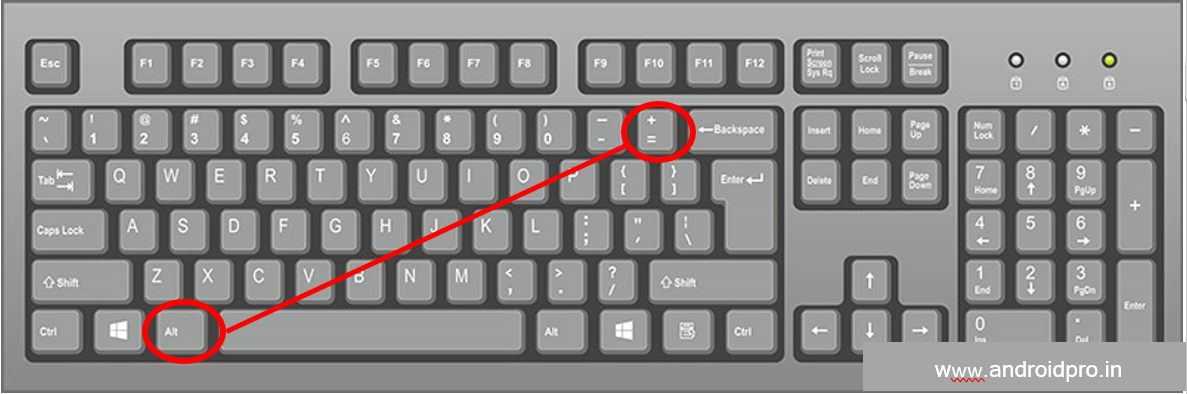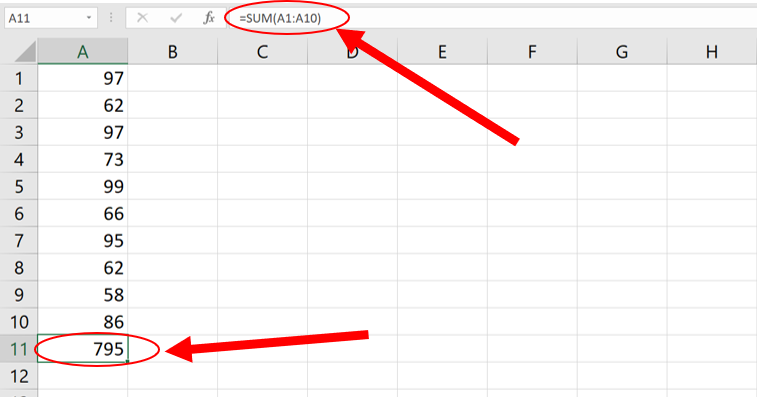# Sum Formula in EXCEL In Excel | Advance Excel

As the word Sum itself means to add means to add more than one number. In exactly the same way, Sum Formula is also used in Excel to add two or more numbers.

3 ways to calculate Addition or sum in MS Excel.

We use the formula of Sum when we have to add numeric type data.

Note: To apply any formula in MS EXCEL, we have to start with Equals = sign only and it is mandatory.

Syntax : =Sum(Number 1, Number 2, . . . . )

#### 1 Sum Using Number:

Example =sum(4,5,6)

### #2Method AUTOSUM In Excel ALT + =In this method, we will learn how to find the sum or total using the shortcut key, the keyboard is even easier, you just have to press the ALT + = key together from your keyboard, and as soon as you press it, The formula of sum will be automatically applied in that cell which will be kept active. As can be seen in the image below.